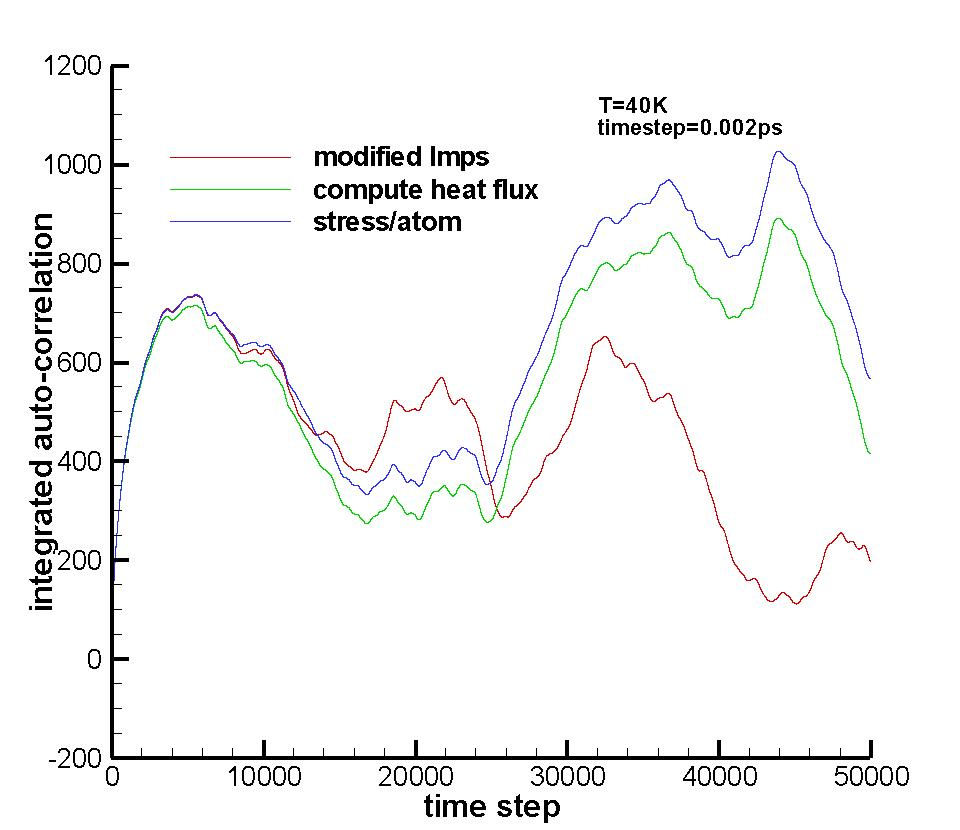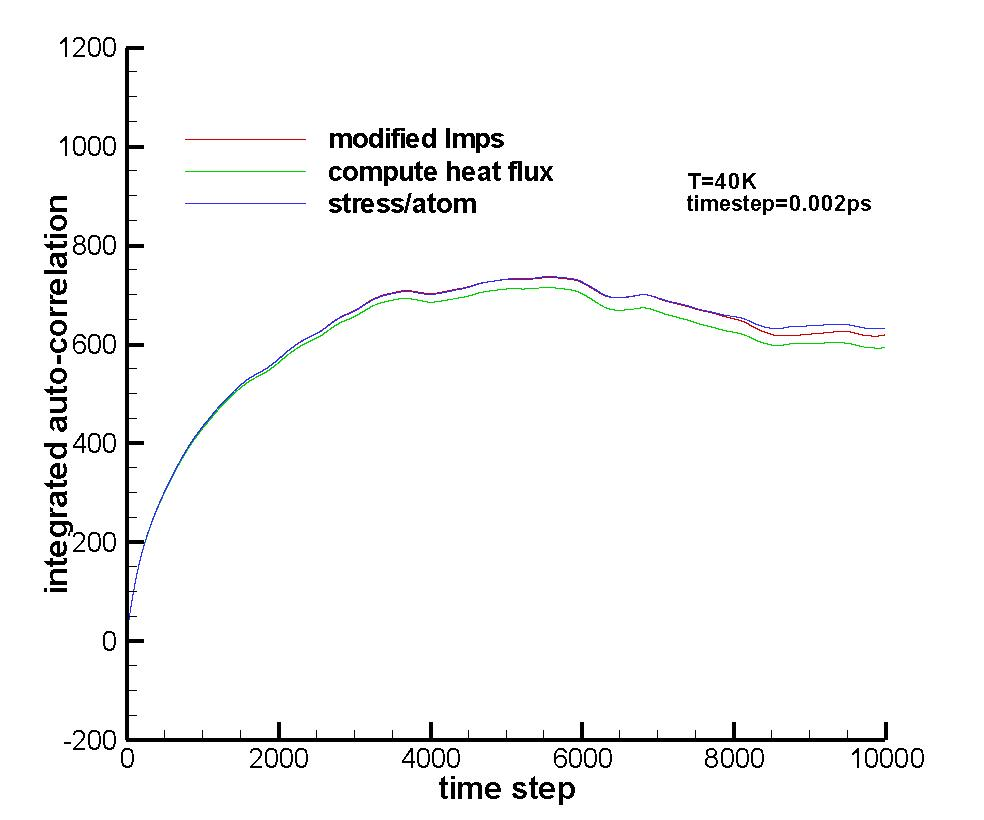# [lammps-users] modification to potential subroutines to use Green-Kubo thermal conductivity calculation

Dear all:

I have gone through the compute heat flux thread by German and other users, and I found that by using the stress/atom, the original compute heat flux command is obsolete. For some reason, I need to modify the source code to compute the heat flux by myself. I started with the pair_lj_cut.cpp and calculated the heat flux (output to a separate file) due to the LJ potential and combine it with the convection term to obtain the total flux. The following is a part of the modified pair_lj_cut.cpp. The complete file is also attached. Also attached is the comparison of integrated autocorrelation function of heat flux. At the beginning, the modified lmps gives very close results to stress/atom method. However, the divergence is serious as time goes on. Is the velocities called in in pair_lj_cut.cpp is not the correct velocity to be used? or it’s just the numerical error?

//----TENGFEI MOD.

//CALCULATE VIRAL HEAT FLUX DUE TO PAIR INTERACTION

fv_ljc=delxfpair(velo[i]+velo[j]);

fv_ljc=delyfpair(velo[i]+velo[j]);

fv_ljc=delzfpair(velo[i]+velo[j]);

qflux2_ljc+=delx*(fv_ljc+fv_ljc+fv_ljc);

qflux2_ljc+=dely*(fv_ljc+fv_ljc+fv_ljc);

qflux2_ljc+=delz*(fv_ljc+fv_ljc+fv_ljc);

//TENGFEI MOD.----

if (eflag) {

evdwl = r6inv*(lj3[itype][jtype]*r6inv-lj4[itype][jtype]) -

offset[itype][jtype];

evdwl *= factor_lj;

}

if (evflag) ev_tally(i,j,nlocal,newton_pair,

evdwl,0.0,fpair,delx,dely,delz);

}

}

}

//----TENGFEI MOD.

//PRINT HEAT FLUX TO FILE “bond_flux”

MPI_Comm_rank(world,&me);

if (me==0){ if (nstep_ljc==1) ljc_flux=fopen(“ljc_flux”,“w”);

}

//gather all answers from other processes

MPI_Reduce(&qflux2_ljc, &answer, 3, MPI_DOUBLE, MPI_SUM, 0, world);

MPI_Comm_rank(world,&me);

//write flux to file

//TENGFEI MOD.----

I hope I haven’t missed anything, more information will be provided if needed.

I appreciate any comment!

Tengfei

pair_lj_cut.cpp (22.2 KB)in.lj.compute (879 Bytes)

in.lj.mod (1.04 KB)

in.lj.script (1.69 KB)